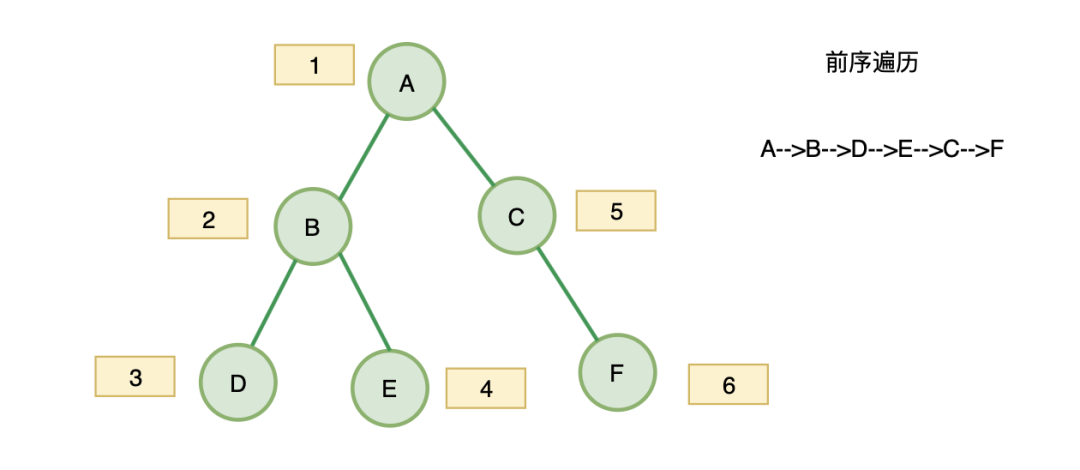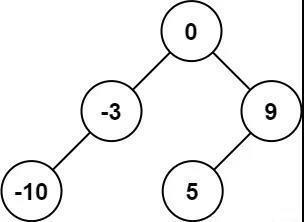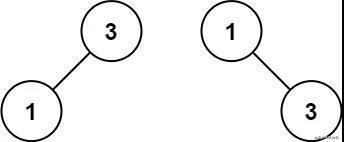# leetcode 最常见的前端基础算法面试题（上）## 二叉树（DFS）

### 二叉树前中后遍历套路详解var Traversal = function(root) {    const stack = [];    while (root || stack.length){      while(root){        stack.push(root);        root = root.left;      }      root = stack.pop();      root = root.right;    }    return res;};

• A、B、D 入栈，

• D 出栈

• B 出栈

• E 入栈

• E 出栈

• A 出栈

• C 入栈

• C 出栈

• F 入栈

• F 出栈

var preorderTraversal = function(root) {    // 初始化数据    const res =[];    const stack = [];    while (root || stack.length){      while(root){        res.push(root.val);        stack.push(root);        root = root.left;      }      root = stack.pop();      root = root.right;    }    return res;};var preorderTraversal = function(root) {    // 初始化数据    const res =[];    const stack = [];    while (root || stack.length){      while(root){        stack.push(root);        root = root.left;      }      root = stack.pop();      res.push(root.val);      root = root.right;    }    return res;};var postorderTraversal = function(root) {  // 初始化数据    const res =[];    const stack = [];    while (root || stack.length){      while(root){        stack.push(root);        res.unshift(root.val);        root = root.right;      }      root = stack.pop();      root = root.left;    }    return res;};

### 对称二叉树

   1   / \  2   2 / \ / \3  4 4  3

1   / \  2   2   \   \   3    3

• 判断两个指针当前节点值是否相等

• 判断 A 的右子树与 B 的左子树是否对称

• 判断 A 的左子树与 B 的右子树是否对称

function isSame(leftNode, rightNode){    if(leftNode === null && rightNode === null) return true;    if(leftNode === null || rightNode === null) return false;    return leftNode.val === rightNode.val && isSame(leftNode.left, rightNode.right) && isSame(leftNode.right, rightNode.left)}var isSymmetric = function(root) {    if(!root) return root;    return isSame(root.left, root.right);};

### 二叉树的最大深度

• 只要遍历到这个节点既没有左子树，又没有右子树的时候

• 说明就到底部了，这个时候如果之前记录了深度，就可以比较是否比之前记录的深度大，大就更新深度

• 然后以此类推，一直比较到深度最大的

var maxDepth = function(root) {    if(!root) return root;    let ret = 1;    function dfs(root, depth){        if(!root.left && !root.right) ret = Math.max(ret, depth);        if(root.left) dfs(root.left, depth+1);        if(root.right) dfs(root.right, depth+1);    }    dfs(root, ret);    return ret};

### 将有序数组转化为二叉搜索树输入：nums = [-10,-3,0,5,9]输出：[0,-3,9,-10,null,5]解释：[0,-10,5,null,-3,null,9] 也将被视为正确答案：输入：nums = [1,3]输出：[3,1]解释：[1,3] 和 [3,1] 都是高度平衡二叉搜索树。 提示：1 <= nums.length <= 104-104 <= nums[i] <= 104nums 按 严格递增 顺序排列

• 构建一颗树包括：构建 root、构建 root.left 和 root.right

• 题目要求"高度平衡" — 构建 root 时候，选择数组的中间元素作为 root 节点值，即可保持平衡。

• 递归函数可以传递数组，也可以传递指针，选择传递指针的时候：l r 分别代表参与构建 BST 的数组的首尾索引。

var sortedArrayToBST = function(nums) {    return toBST(nums, 0, nums.length - 1)};const toBST = function(nums, l, r){    if( l > r){        return null;    }    const mid = l + r >> 1;    const root = new TreeNode(nums[mid]);    root.left = toBST(nums, l, mid - 1);    root.right = toBST(nums, mid + 1, r);    return root;}

## 栈

### 有效的括号

示例 1：输入：s = "()"输出：true示例 2：输入：s = "()[]{}"输出：true示例 3：输入：s = "(]"输出：false示例 4：输入：s = "([)]"输出：false

var isValid = function(s) {    const map = { '{': '}', '(': ')', '[': ']' };    const stack = [];    for(let i of s){        if(map[i]){            stack.push(i);        } else {            if(map[stack[stack.length - 1]] === i){                stack.pop()            }else{                return false;            }        }    }    return stack.length === 0;};

### 最小栈

• push(x) —— 将元素 x 推入栈中。

• pop() —— 删除栈顶的元素。

• top() —— 获取栈顶元素。

• getMin() —— 检索栈中的最小元素。

示例:MinStack minStack = new MinStack();minStack.push(-2);minStack.push(0);minStack.push(-3);minStack.getMin();   --> 返回 -3.minStack.pop();minStack.top();      --> 返回 0.minStack.getMin();   --> 返回 -2.提示：pop、top 和 getMin 操作总是在 非空栈 上调用。

var MinStack = function() {    this.stack = [];};MinStack.prototype.push = function(x) {    this.stack.push(x);};MinStack.prototype.pop = function() {    this.stack.pop();};MinStack.prototype.top = function() {    return this.stack[this.stack.length - 1];};

• 开始我们向 stack push -2

• 此时辅助栈 minStack，因为此时 stack 最小的是-2，也 push -2stack push 0

• 此时辅助站 minStack 会用 0 跟 -2 对比，-2 更小，minstack 会 push -2

• stack push -3

• 此时辅助站 minStack 会用 -3 跟 -2 对比，-3 更小，minstack 会 push -3

var MinStack = function() {    this.stack = [];    // 辅助栈    this.minStack = [];};MinStack.prototype.push = function(x) {    this.stack.push(x);    // 如果是第一次或者当前x比最小栈里的最小值还小才push x    if(this.minStack.length === 0 || x < this.minStack[this.minStack.length - 1]){        this.minStack.push(x)    } else {         this.minStack.push( this.minStack[this.minStack.length - 1])    }};MinStack.prototype.pop = function() {    this.stack.pop();    this.minStack.pop();};MinStack.prototype.top = function() {    return this.stack[this.stack.length - 1];};MinStack.prototype.getMin = function() {    return this.minStack[this.stack.length - 1];};

## 动态规划

### 最大子序和

示例 1：输入：nums = [-2,1,-3,4,-1,2,1,-5,4]输出：6解释：连续子数组 [4,-1,2,1] 的和最大，为 6 。示例 2：输入：nums = 输出：1示例 3：输入：nums = 输出：0

• 这道题可以用动态规划来解决，关键是找动态转移方程

• 我们动态转移方程中，dp 表示每一个 nums 下标的最大自序和，所以 dp[i]的意思为：包括下标 i 之前的最大连续子序列和为 dp[i]。

dp[i]只有两个方向可以推出来：

• 1、如果 dp[i - 1] < 0，也就是当前遍历到 nums 的 i，之前的最大子序和是负数，那么我们就没必要继续加它了，因为 dp[i] = dp[i - 1] + nums[i] 会比 nums[i]更小，所以此时还不如 dp[i] = nums[i]，就是目前遍历到 i 的最大子序和呢

• 2、同理 dp[i - 1] > 0，说明 nums[i]值得去加 dp[i - 1]，此时回避 nums[i]更大

var maxSubArray = function(nums) {  let res = nums;  const dp = [nums];  for(let i=1;i < nums.length;i++){      if(dp[i-1]>0){        dp[i]=nums[i]+dp[i-1]      }else{       dp[i]=nums[i]      }          res=Math.max(dp[i],res)  }    return res};

### 爬楼梯

示例 1：输入： 2输出： 2解释： 有两种方法可以爬到楼顶。1.  1 阶 + 1 阶2.  2 阶示例 2：输入： 3输出： 3解释： 有三种方法可以爬到楼顶。1.  1 阶 + 1 阶 + 1 阶2.  1 阶 + 2 阶3.  2 阶 + 1 阶

var climbStairs = function(n) {    const dp = {};    dp = 1;    dp = 2;    for(let i = 3; i <= n; i++){        dp[i] = dp[i-1] + dp[i-2]    }    return dp[n]};## 评论